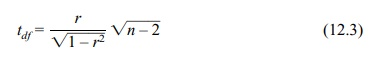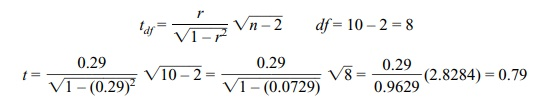# Testing Hypotheses about the Correlation Coefficient

| Home | | Advanced Mathematics |

## Chapter: Biostatistics for the Health Sciences: Correlation, Linear Regression, and Logistic Regression

In addition to assessing the strength of association between two variables, we need to know whether their association is statistically significant.

TESTING HYPOTHESES ABOUT THE CORRELATION COEFFICIENT

In addition to assessing the strength of association between two variables, we need to know whether their association is statistically significant. The test for the significance of a correlation coefficient is based on a t test. In Section 12.4, we presented r (the sample statistic for correlation) and ρ (the population parameter for the correlation between X and Y in the population).

The test for the significance of a correlation evaluates the null hypothesis (H0) that ρ = 0 in the population. We assume Y = α + bx + ε. Testing ρ = 0 is the same as testing b = 0. The term ε in the equation is called the noise term or error term. It is also sometimes referred to as the residual term. The assumption required for hypothesis testing is that the noise term has a normal distribution with a mean of zero and unknown variance σ2 independent of X. The significance test for Pearson’s correlation coefficient iswhere df = n – 2; n = number of pairs.

Referring to the earlier example presented in Table 12.2, we may test whether the previously obtained correlation is significant by using the following procedure:where p = n.s., t critical = 2.306, 2-tailed.